Example Questions

Example Question #20 : Equations / Inequalities

Satoshi and Reginald are bottlecap collectors, and Satoshi has 46 more bottlecaps than Reginald. If they were both to receive 6 bottle caps each, Satoshi would have three times as many bottle caps as Reginald. How many bottlecaps does Satoshi have?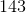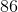Explanation:

The mathematical approach to this problem would be to set up a system of equations; one which represents the current relationship of Satoshi's and Reginald's number of bottle caps: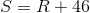And one which represents the relationship 6 bottle caps later, wherein Satoshi would have three times as many bottlecaps as Reginald: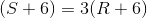Subtracting the first equation from the second equation provides: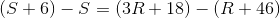Which simplifies to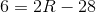Solving this yields the value for Reginald's number of bottle caps, which is 17, which can be plugged back into the very first equation to find Satoshi's number of bottle caps, which is 63.

There is, however, another way to find this solution, which is by first looking at the answer choices.

Since we know that Satoshi's number of bottlecaps plus six must be divisible by 3 (since it is three times Reginald's new number of caps), we can quickly eliminate the choices 86, 143, and 52. Furthermore, since Satoshi has 46 more bottlecaps than Reginald, it'd be impossible for him to have 21 bottlecaps.  This leaves only the 63 option.

When taking standardized math tests, where time management is key, it can be helpful to look for quicker ways to find a solution, such as back-solving, rather than just solving the problem normally.

Example Question #21 : Equations / Inequalities

Axel is traveling a distance of 120 miles to visit a relative in Phoenix. For the first half of his trip, he's traveled at a speed of 40 miles per hour. How fast will he need to drive from that point on in order to average a speed of 60 miles per hour?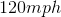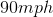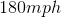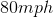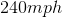Explanation:

To solve this problem, begin by calculating the amount of time it would take to complete the 120 mile journey at an average of 60 miles per hour: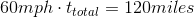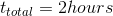Next, calculate the time it would take to complete half of the journey, 60 miles, at a speed of 40 miles per hour.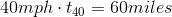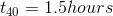In order to average 60 miles per hour, the rest of the journey would need to be completed in the remaining .05 hours. Thus the speed for the final leg of the journey is determined as follows: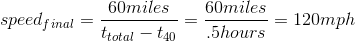Well over the speed limit.

Example Question #22 : Equations / Inequalities

If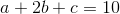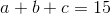, and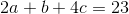, what is the value of?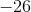The answer cannot be determined from the information given.Explanation:

This problem has three unknowns,,, and,; however, it also has three separate equations which, if they are independent, enable us to solve for these variables.

One way to begin is by solving forusing the first two equations:Subtracting the bottom equation from the top gives: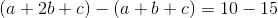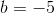The next step will then be to try to combine equations in a way that will eliminate, so that we're only working with our variable of interest,, and, which is now known. To do this, let's compare the second and third equations:In order to eliminate the, we will want it to have the same coefficient in both equations. We can do this by multiplying the top equation by 4 to get a new system of equations: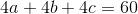Subtracting the top from the bottom yields: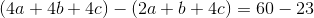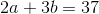Plugging in the value forthen gives: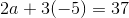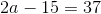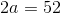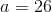Example Question #21 : Linear / Rational / Variable Equations

What is the value of (5 + x)(10 – y) when x = 3 and y = –3?

104

108

38

56

104

Explanation:

This is a simple plug-in and PEMDAS problem. First, plug in x = 3 and y = –3 into the x and y. You should follow the orders of operation and compute what is within the parentheses first and then find the product. This gives 8 * 13 = 104. The answer is 104.

Example Question #51 : Algebra

If x = 4, and y = 3x + 5, then 2y – 1 equals

47

15

33

22

33

Explanation:

Start by plugging in x = 4 to solve for y: y = 3 * 4 + 5 = 17.  Then 2 * 17 – 1 = 33

Example Question #1831 : Act Math

Sarah’s current age is three times Ron’s age two years ago. Sarah is currently 14 years older than Ron. What is the sum of Sarah and Ron’s current age?

36

34

24

32

34

Explanation:

The best way to solve this problem is to turn the two statements into equations calling Sarah’s age S and Ron’s age R. So, S = 3(R – 2) and S = 14 + R. Now substitute the value for S in the second equation for the value of S in the first equation to get 14 + R = 3(R – 2) and solve for R. So R equals 10 so S equals 24 and the sum of 10 and 24 is 34.

Example Question #26 : Equations / Inequalities

A store sells potatoes for $0.24 and tomatoes for$0.76. Fred bought 12 individual vegetables. If he paid \$6.52 total, how many potatoes did Fred buy?

5

7

2

8

5

Explanation:

Set up an equation to represent the total cost in cents: 24P + 76T = 652. In order to reduce the number of variables from 2 to 1, let the # tomatoes = 12 – # of potatoes. This makes the equation 24P + 76(12 – P) = 652.

Solving for P will give the answer.

Example Question #61 : Linear / Rational / Variable Equations

Kim is twice as old as Claire. Nick is 3 years older than Claire. Kim is 6 years older than Emily. Their ages combined equal 81. How old is Nick?

22

17

13

27

17

Explanation:

The goal in this problem is to have only one variable. Variable “x” can designate Claire’s age.

Then Nick is x + 3, Kim is 2x, and Emily is 2x – 6; therefore x + x + 3 + 2x + 2x – 6 = 81

Solving for x gives Claire’s age, which can be used to find Nick’s age.

Example Question #22 : Linear / Rational / Variable Equations

If 6h – 2g = 4g + 3h

In terms of g, h = ?

g

5g

3g

4g

2g

2g

Explanation:

If we solve the equation for b, we add 2g to, and subtract 3h from, both sides, leaving 3h = 6g. Solving for h we find that h = 2g.

Example Question #1 : How To Find The Solution To An Equation

If 2x + y = 9 and y – z = 4 then 2x + z = ?

13

Cannot be determined

5

21

29

5

Explanation:

If we solve the first equation for 2x we find that 2x = 9 – y. If we solve the second equation for z we find z = –4 + y. Adding these two manipulated equations together we see (2x) + (y) = (9 – y)+(–4 + y).

The y’s cancel leaving us with an answer of 5.

Tired of practice problems?

Try live online GRE prep today.